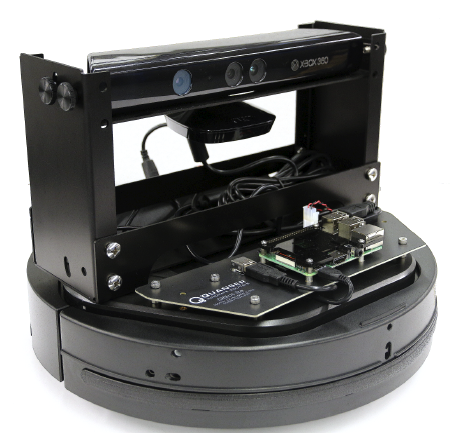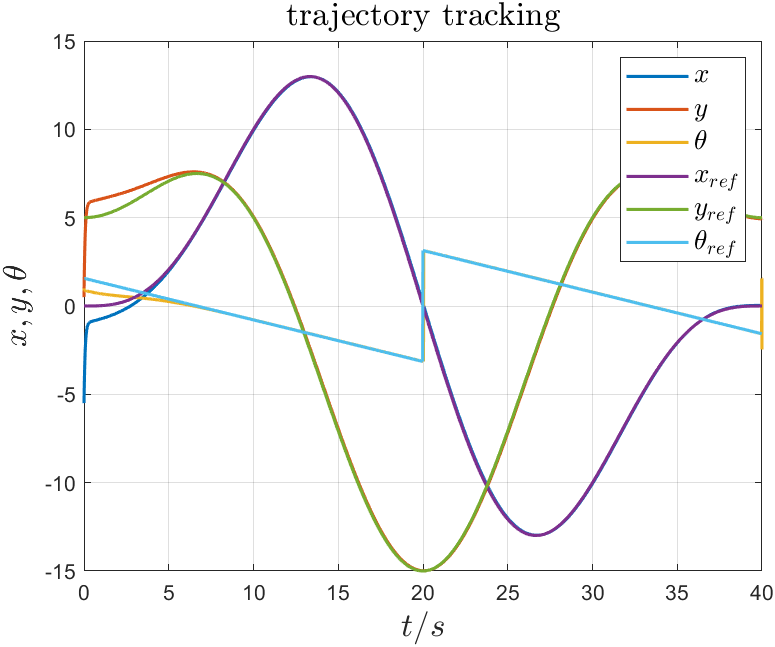0

# 硬核，机器人只需5秒就跟踪上了爱心曲线

🔥 作者：FrigidWinter

🔥 简介：主攻机器人与人工智能领域的理论研究和工程应用，业余丰富各种技术栈。主要涉足：【机器人(ROS)】【机器学习】【深度学习】【计算机视觉】

🔥 专栏

• 《机器人原理与技术》
• 《计算机视觉教程》
• 《机器学习》
• 《嵌入式系统》

## 0 前言## 2.1 选择轨迹

``````R =5.0;
T =40.0;
w =2 * pi / T;

ref = @(t)[R*(2*sin(w*t)-sin(2*w*t)), R*(2*cos(w*t)-cos(2*w*t))];
``````## 2.2 编写轨迹跟踪算法

``````function dpdt = wmrTrackOde45Func(t, p, ref)
``````

``````% robot state
x = p(1);
y = p(2);
theta = p(3);
``````

``````% reference path
pRef = ref(t);
xRef = pRef(1);
yRef = pRef(2);
vRef =1;
wRef =0;
``````

``````% predict reference path
targetVec =[xRef - x;
yRef - y];if targetVec(2)>0
thetaRef = acos(targetVec(1) / sqrt(targetVec(1)^2 + targetVec(2)^2));else
thetaRef = - acos(targetVec(1) / sqrt(targetVec(1)^2 + targetVec(2)^2));
end
``````

``````% controller parameters
Kx =15;
Ky =25;
Kt =10;
``````

``````% controller
ex = cos(theta) * (xRef - x) + sin(theta) * (yRef - y);
ey = -sin(theta) * (xRef - x) + cos(theta) * (yRef - y);
u  =[ vRef * cos(thetaRef - theta) + Kx * ex;
wRef + vRef*(Ky * ey + Kt * sin(thetaRef - theta))];v= u(1);
w = u(2);

dpdt =[v*cos(theta); v*sin(theta); w];
end
``````

## 2.3 测试跟踪性能## 2.4 制作动图

``````figure(1)
trackProcess = VideoWriter('video/trackProcess.avi');
open(trackProcess);
movie = moviein(t);

% 画参考轨迹
plot(refPos(:,1),refPos(:,2), "--b", 'LineWidth', 1);
grid on
hold on

% 画初始位置
handler = plotPose(initPos, 0.8, 0.3, 'r', size);fori=1:length(t)
delete(handler);
handler = plotPose(actualPos(i,:));
plot(actualPos(i,1), actualPos(i,2), 'Marker', '.', 'color', [0,0.5,0]);
movie(:, i)= getframe;
writeVideo(trackProcess, movie(:, i));
end
hold off
close(trackProcess);
``````

## 3 真车实战## 4 参考文献

杨士超. 非完整轮式移动机器人的反馈镇定和轨迹跟踪控制研究[D]. 长安大学.
Kanayama Y , Kimura Y , Miyazaki F , et al. A stable tracking control method for an autonomous mobile robot[C]// IEEE International Conference on Robotics & Automation. IEEE, 1991.

### “硬核，机器人只需5秒就跟踪上了爱心曲线”的评论:

##### 关于作者##### overfit同步小助手